### Home > CALC > Chapter 11 > Lesson 11.2.3 > Problem11-74

11-74.
1.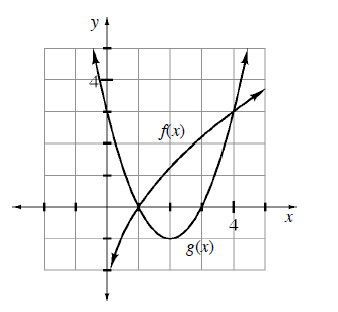Multiple Choice: Which integral below calculates the volume generated when the region bounded by f(x) and g(x) (shown at right) is rotated about the y-axis? Homework Help ✎

1.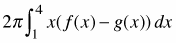2.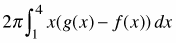3.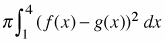4.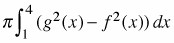5. None of these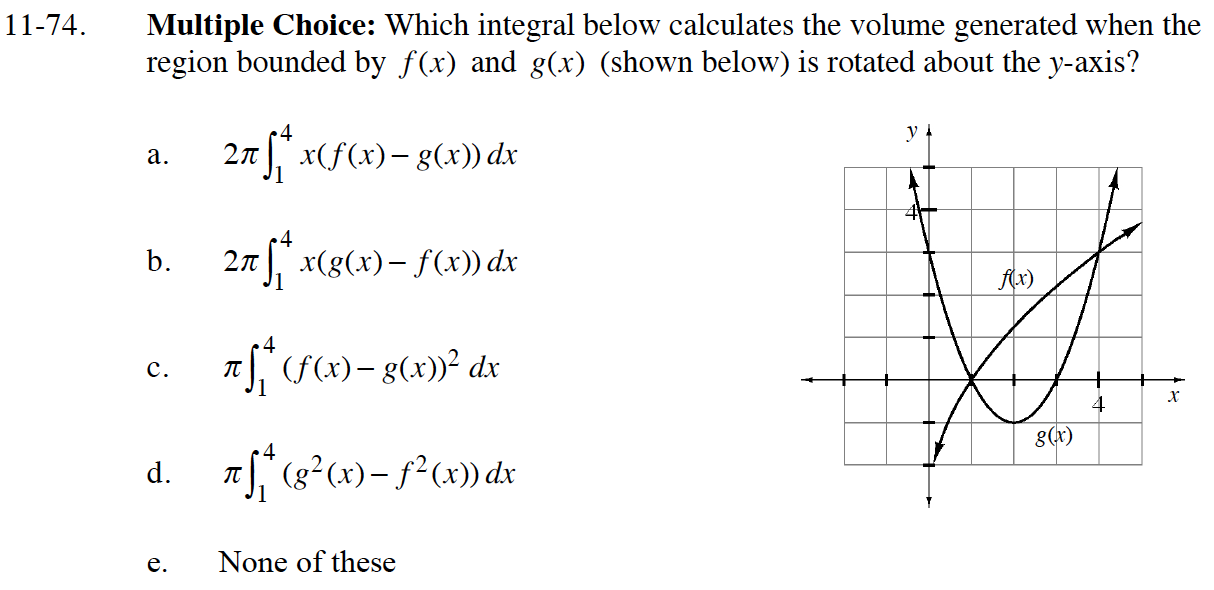This is a situation where it works well to use shells. What is the formula for using shells?